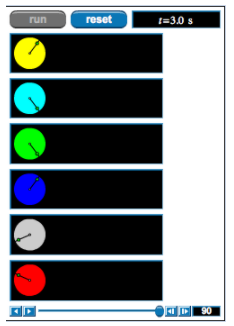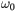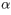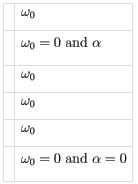# Problem: This applet shows six disks rotating with constant angular acceleration. No two have the same initial angular velocity and angular acceleration. To answer the following questions, number the disks starting from the top. That is, call the yellow disk "1" and go sequentially down to the red disk, which will be "6". In the following questions, you will be asked to determine whether the disks' angular velocities and accelerations are positive, negative, or zero. Keep in mind that angular velocity is considered positive if rotation is in the counterclockwise direction. Angular acceleration is positive if the rotation is in the counterclockwise (positive) direction and the angular speed is increasing, or if rotation is in the clockwise (negative) direction and the angular speed is decreasing (thus the angular velocity is becoming less negative). Negative angular acceleration is defined analogously.Part AWhich of the disks have positive initial angular velocity?Write down the numbers, in order, that correspond to the disk(s) that you believe are correct, without commas or spaces between them. For example, if you think that the yellow disk and the gray disk are the correct ones, then you should enter15.Part BWhich of the disks have negative initial angular velocity?Write down the numbers, in order, that correspond to the disk(s) that you believe are correct, without commas or spaces between them. For example, if you think that the yellow disk and the gray disk are the correct ones, then you should enter15.Part CWhich of the disks have zero angular acceleration?Write down the numbers, in order, that correspond to the disk(s) that you believe are correct, without commas or spaces between them. For example, if you think that the yellow disk and the gray disk are the correct ones, then you should enter15.Part DWhich of the disks have positive angular acceleration?Write down the numbers, in order, that correspond to the disk(s) that you believe are correct, without commas or spaces between them. For example, if you think that the yellow disk and the gray disk are the correct ones, then you should enter15.Part EWhich of the following characterizes the initial angular velocityand the angular accelerationof disk 4?

###### FREE Expert Solution

Part A

The disks have a positive initial velocity if the rotation is in a counterclockwise direction.

87% (98 ratings)###### Problem DetailsThis applet shows six disks rotating with constant angular acceleration. No two have the same initial angular velocity and angular acceleration. To answer the following questions, number the disks starting from the top. That is, call the yellow disk "1" and go sequentially down to the red disk, which will be "6". In the following questions, you will be asked to determine whether the disks' angular velocities and accelerations are positive, negative, or zero. Keep in mind that angular velocity is considered positive if rotation is in the counterclockwise direction. Angular acceleration is positive if the rotation is in the counterclockwise (positive) direction and the angular speed is increasing, or if rotation is in the clockwise (negative) direction and the angular speed is decreasing (thus the angular velocity is becoming less negative). Negative angular acceleration is defined analogously.

Part A

Which of the disks have positive initial angular velocity?

Write down the numbers, in order, that correspond to the disk(s) that you believe are correct, without commas or spaces between them. For example, if you think that the yellow disk and the gray disk are the correct ones, then you should enter15.

Part B

Which of the disks have negative initial angular velocity?

Write down the numbers, in order, that correspond to the disk(s) that you believe are correct, without commas or spaces between them. For example, if you think that the yellow disk and the gray disk are the correct ones, then you should enter15.

Part C

Which of the disks have zero angular acceleration?

Write down the numbers, in order, that correspond to the disk(s) that you believe are correct, without commas or spaces between them. For example, if you think that the yellow disk and the gray disk are the correct ones, then you should enter15.

Part D

Which of the disks have positive angular acceleration?

Write down the numbers, in order, that correspond to the disk(s) that you believe are correct, without commas or spaces between them. For example, if you think that the yellow disk and the gray disk are the correct ones, then you should enter15.

Part E

Which of the following characterizes the initial angular velocityand the angular accelerationof disk 4?